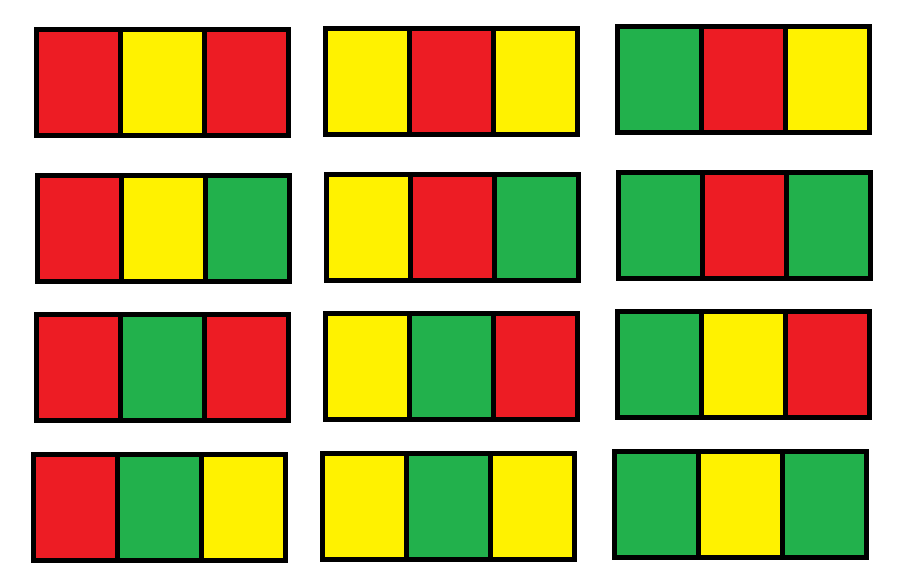Leetcode 1411：给Nx3网格图涂色的方案数（超详细的解法！！！）
in leetcode with 0 comment

输入：n = 1输入：n = 2

输入：n = 3

输入：n = 7

输入：n = 5000

• n == grid.length
• grid[i].length == 3
• 1 <= n <= 5000

from functools import lru_cache
class Solution:
def numOfWays(self, n: int) -> int:
mod = 10 ** 9 + 7
s1 = set([1, 2, 3])

@lru_cache(None)
def dfs(x, y, pre, cur):
s2 = [pre[y]]
if y: s2.append(cur[y - 1])
t = list(s1 - set(s2)) # 当前位置可以使用的颜色

if y == 2:
if x == n - 1: return len(t)
res = 0
for i in t:
t_cur = list(cur)
t_cur[y] = i
res = (res + dfs(x + 1, 0, tuple(t_cur), (0, 0, 0))) % mod
return res

res = 0
for i in t:
t_cur = list(cur)
t_cur[y] = i
res = (res + dfs(x, y + 1, pre, tuple(t_cur))) % mod
return res

return dfs(0, 0, (0, 0, 0), (0, 0, 0))

• $f_{aba}(n)=3*f_{aba}(n-1)+2*f_{abc}(n-1)$
• $f_{abc}(n)=2*f_{aba}(n-1)+2*f_{abc}(n-1)$

class Solution:
def numOfWays(self, n: int) -> int:
aba, abc, mod = 6, 6, 10**9 + 7
for i in range(n - 1):
aba, abc = aba * 3 + abc * 2, aba * 2 + abc * 2
return (aba + abc) % mod

reference:

https://leetcode.com/problems/number-of-ways-to-paint-n-3-grid/discuss/574923/JavaC%2B%2BPython-DP-O(1)-Space

「如果我的文章对你有很大帮助，那么不妨～！」Responses